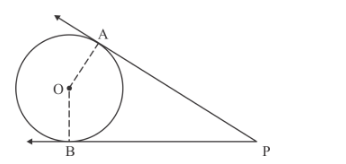# Two tangents PA and PB are drawn from`
Question:

Two tangents PA and PB are drawn from an external point P to a circle with centre O. Prove that AOBP is a cyclic quadrilateral.

Solution:

We are given two tangents PA and PB drawn to a circle with centre from external point P

We are to prove that quadrilateral AOBP is cyclicWe know that tangent at a point to a circle is perpendicular to the radius through that point.

Therefore from figure

$O A \perp A P$

$O B \perp B P$

That is

$\angle O A P=90^{\circ}$

$\angle O B P=90^{\circ}$

$\angle O A P+\angle O B P=90^{\circ}+90^{\circ}$

$=180^{\circ}$.........(1)

$\angle O A P+\angle O B P+\angle A P B+\angle A O B=360^{\circ}$ [Sum of angles of a quadrilateral $=360^{\circ}$ ]

$180^{\circ}+\angle A P B+\angle A O B=360^{\circ} \quad[$ From $(1)]$

$\angle A P B+\angle A O B=360^{\circ}-180^{\circ}$

$\angle A P B+\angle A O B=180^{\circ}$

We know that the sum of opposite angles of cyclic quadrilateral = 180°

Therefore from (1) and (2)